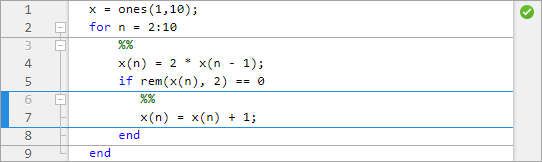## 创建和运行代码节

MATLAB® 代码文件通常包含许多命令和文本行。您通常一次仅专注于代码中的一个部分，分块操作代码和相关文本。要更轻松地进行文档管理和导航，请将您的文件划分为多个节。然后，您可以在单个节中运行代码，并根据需要在各节之间导航。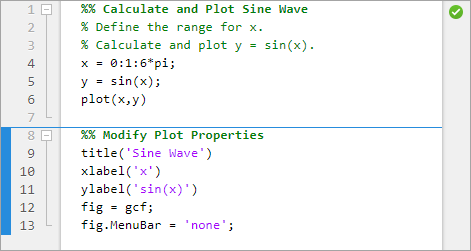### 将文件划分为不同节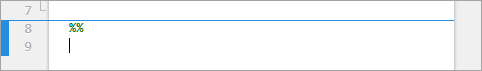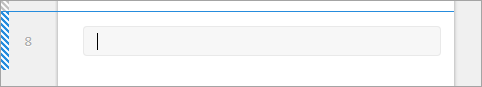### 运行节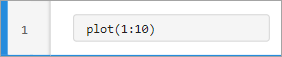#### 递增节中的值

```x = 5; y = factorial(x)```
```y = 120```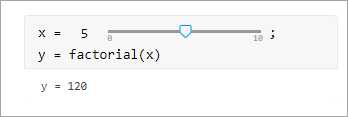### 循环和条件语句中各节的行为

```x = ones(1,10); for n = 2:10 x(n) = 2 * x(n - 1); if rem(x(n), 2) == 0 x(n) = x(n) + 1; end end```

• 在嵌套的最外层，一个节跨整个文件。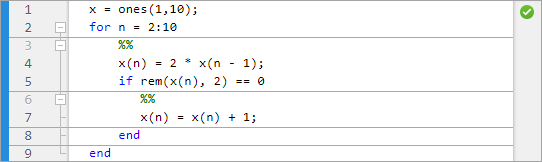• 在嵌套的第二层，一个节存在于 `for` 循环中。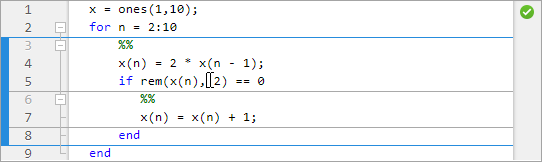• 在嵌套的第三层，一个节存在于 `if` 语句中。